﻿ 5. Detailed description of the Actions > 5.25. Output Actions > 5.25.12. XML File Writer

# 5.25.12. XML File Writer

Icon: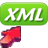Function: writeXML

Property window: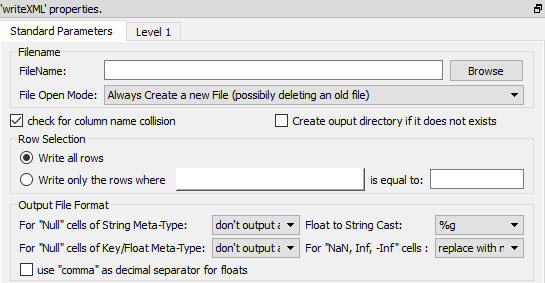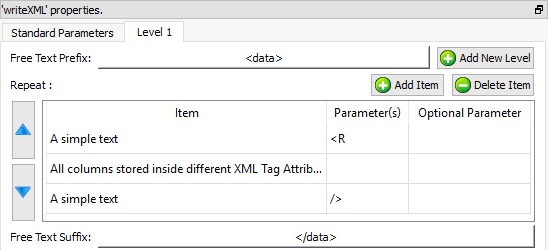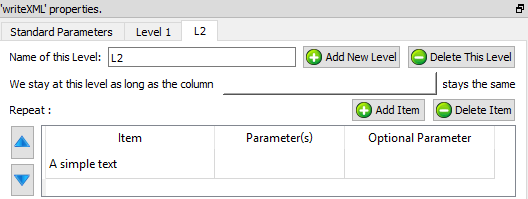Short description:

Create a XML file that contains a table coming from the Anatella transformation graph.

Long Description:

Create a XML file that contains a table coming from the Anatella transformation graph.

Please refer to section 5.1.1 to have more information on how to specify the filename of the XML file (i.e. You can use relative path and Javascript to specify your filename).

The maximum memory consumption of the writeJSON action is equal to the memory required to store one row of the input table. This means that you can create any JSON file, of any size regardless of the quantity of RAM of your server.

The default settings are producing a simple “one-level” XML file (you’ll find more information on the “level” principle below) that represents the input table in a quite straight forward way.

Here are some examples: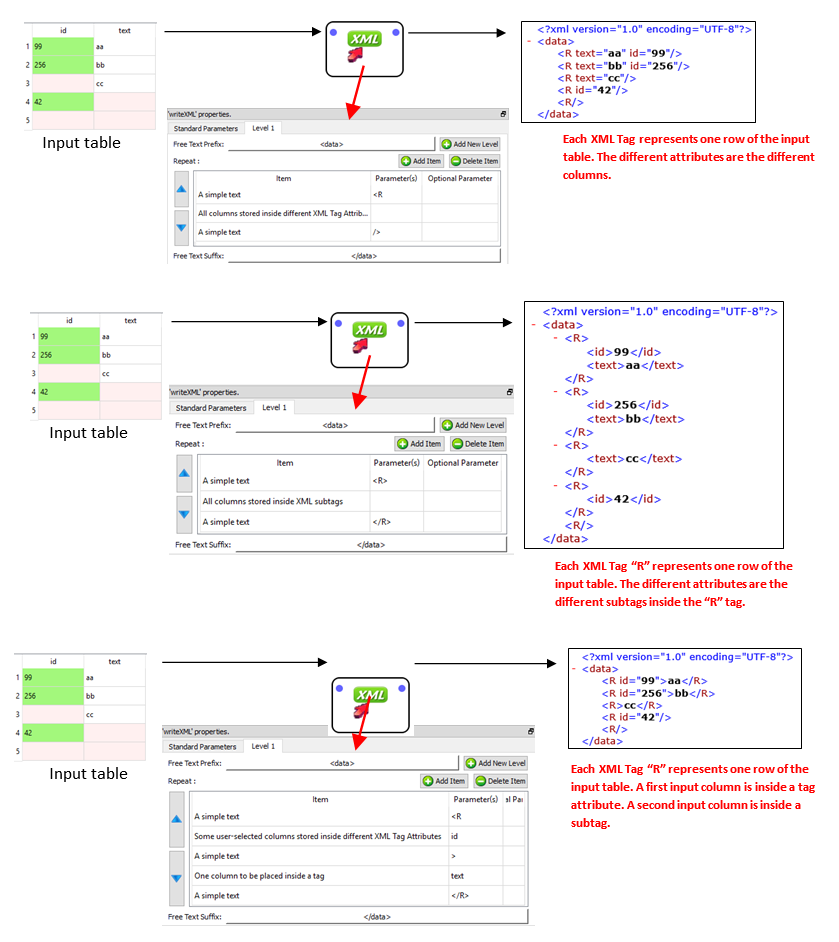Let’s now assume that you have a XML file that has a 2-level structure:

The first, top level contains informations about different customers.

The second, bottom level contains informations about the purchases of each of the customer.

For example, we’ll have something like this: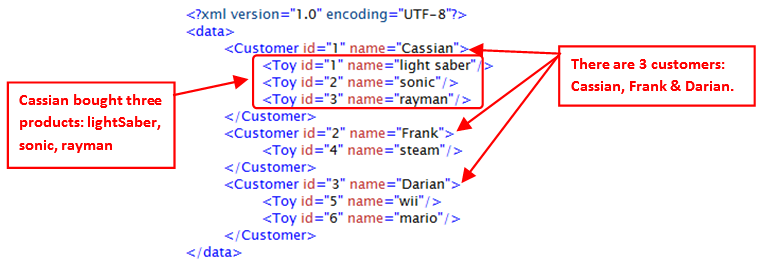The data illustrated in the above XML file is typically initially stored inside two tables (e.g. a “Customers” table and a “Purchases” table) inside a database. To create the above XML file, we’ll use the Join Action to join these two tables and simply forward the output table inside the writeXML action: See illustration below: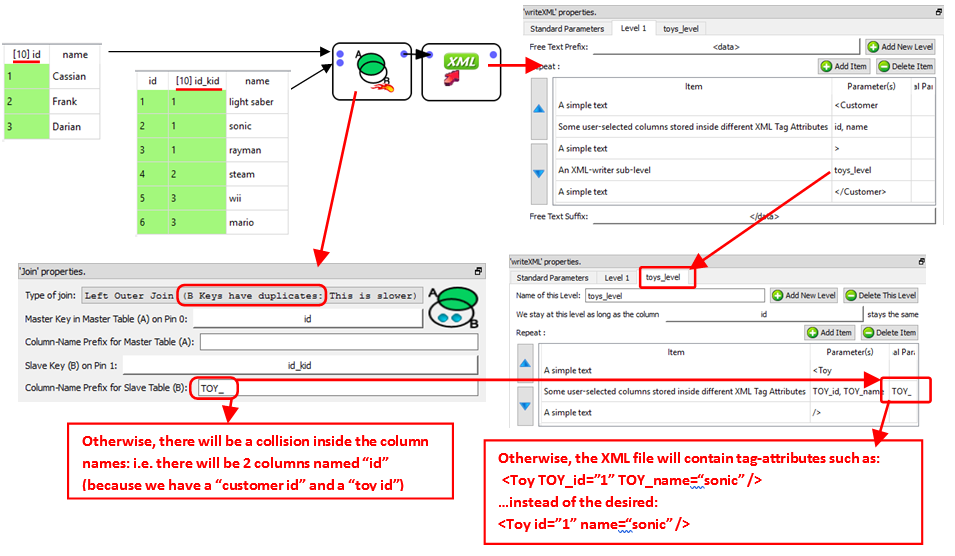The above example shows you how to create a two-level XML file (i.e. the two levels are “Customers” and “Purchases”). Inside Anatella, you can create any XML files with any number of imbricated levels.

It can also happen that a specific level contains several different sub-levels: For example, consider the following XML file: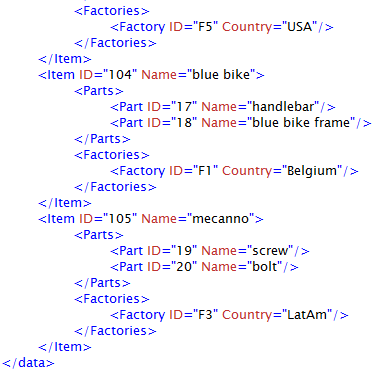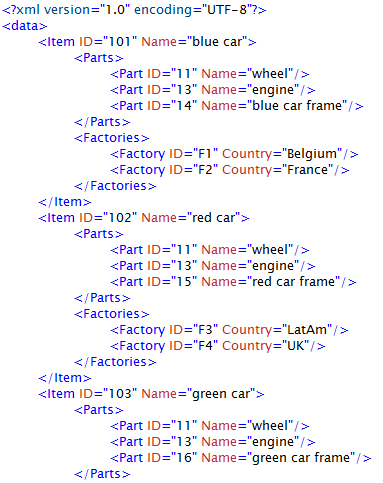The above XML file contains data about different Items (i.e. the first level is about “Items”).

Each item is :

composed of different parts

produced in different Factories.

There are thus two different second-level data: One sub-level is about “Parts” and the other is about “Factories”. Typically, the data illustrated in the above XML file is initially stored inside (at least) three tables (e.g. an “Items” table, a “Parts” table and a “Factories” table) inside a database. To create the above XML file, we’ll join these three tables and simply forward the output table inside the writeXML action: See the illustration below: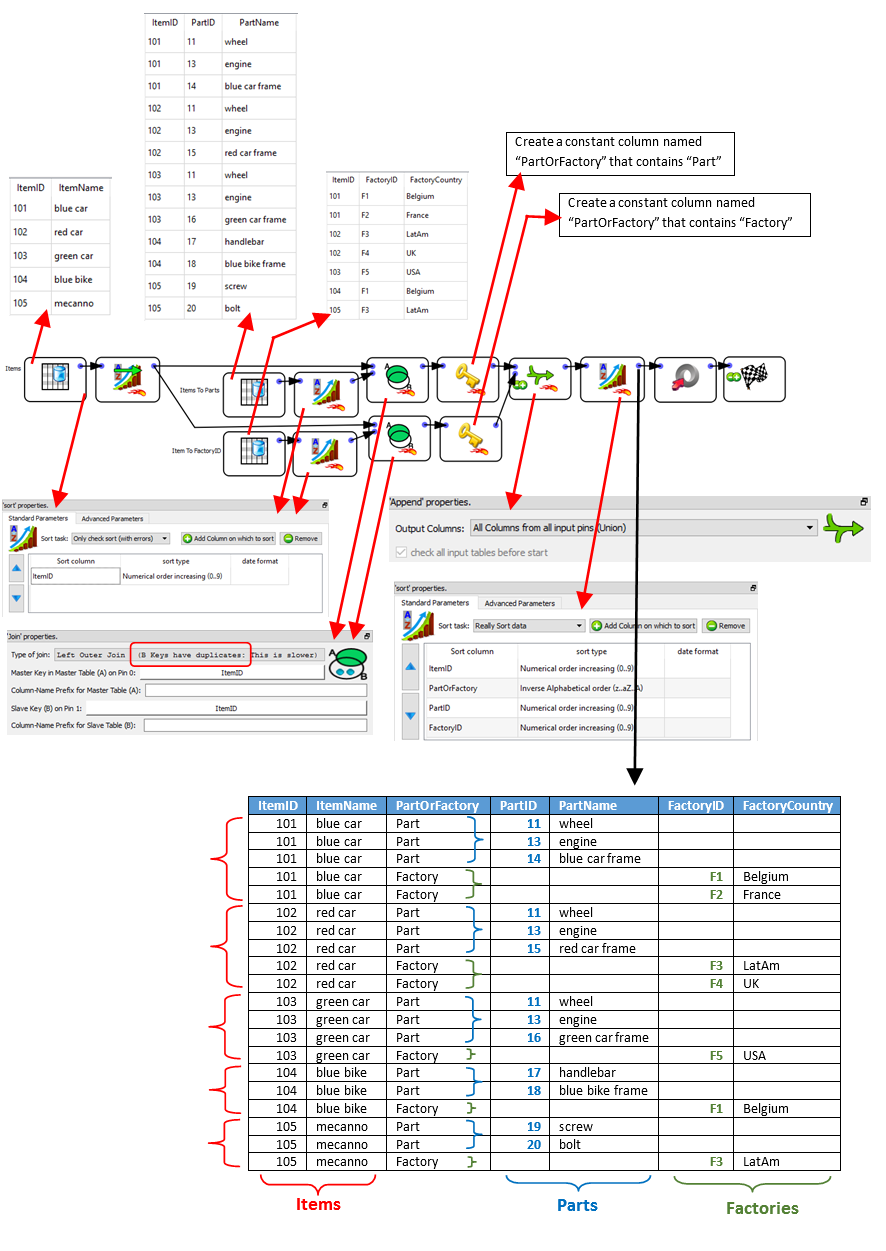The parameters of thewriteXML action are:

The “Level 1” is actually almost empty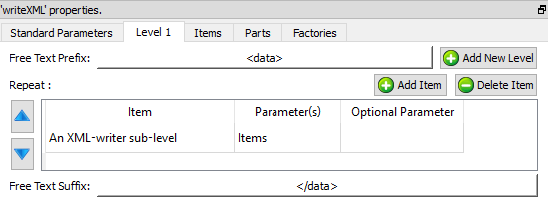The “Item” level is composed of two sub-levels “Parts” and “Factories”. The order is important: first “Parts”, second “Factories”: i.e. the order must match the order used inside the input table: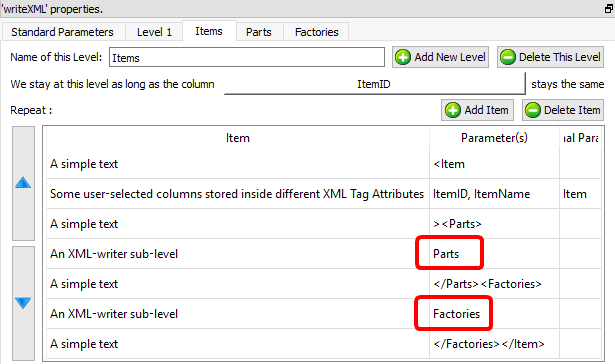The “Parts” level and the “Factories” level are straight forward: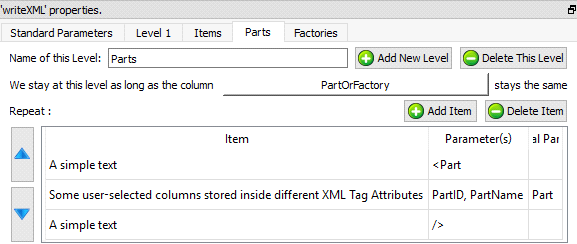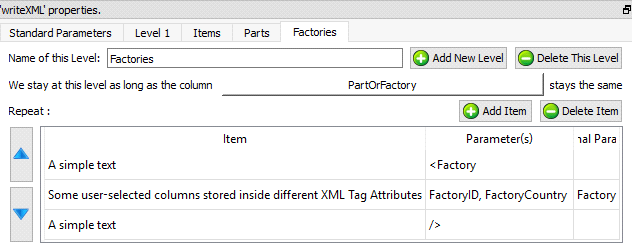You now know how to create any XML file with any structure and of any size (i.e. the RAM memory consumption of thewriteXML action is only the memory required to store ONE row of the input table).

We’ll now introduce a small trick to reduce computation time.

Let’s assume that we want to create the following XML file: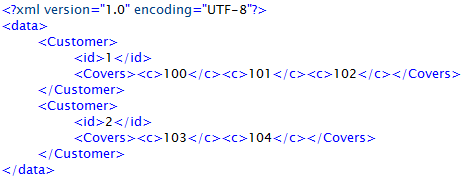To create the above XML file, we can use 2 techniques. Here is the first one (that follows the general principles already explained here above):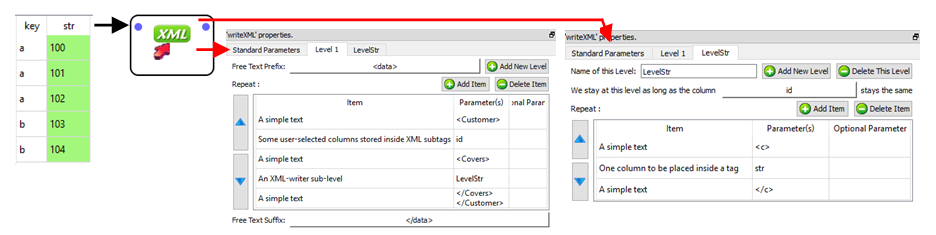…and here is the second technique: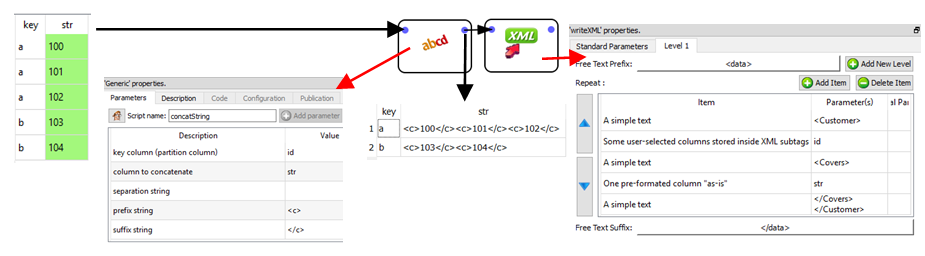The advantages of the second technique are:

the input table to thewriteXML action (i.e. the table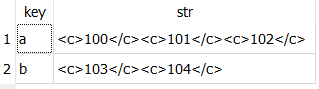) has only 2 rows (compared to 5 rows for the first technique). In general, manipulating tables that contain a smaller quantity of rows leads to a reduced computing time. This is particularly true if the rows are very long (i.e. one thousand columns). The input table in the above example has only a limited number of columns (less than 300), thus the computing-time gain will be inexistent.

the input table to thewriteXML action consumes less disk space since there are less un-needed repetitions. For example, you can see that, for the first technique, the key “a” is repeated 3 times while, for the second technique, the key “a” only appears one time.

The disadvantages of the second technique are:

We were forced to use the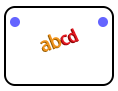concatString Action: This action is very slow because it involves concatenating a large quantity of (possibly very large) strings (and concatenating many strings is always a slow operation). One trick to lessen the impact of this slow speed is to put theconcatString Action inside a N-Way multithreaded section.

The second technique only works for very simple XML files where we don’t have many different imbricated levels inside the XML file.

When using the second technique, we create a row that consumes a large quantity of RAM memory (since the row contains a cell that is the concatenation of all the data required to write the level-2 for a particular level-1). The second technique has thus a quite large memory consumption and it can possibly lead to crashes if the XML file is too big.